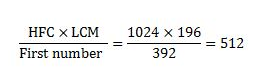# RRB ALP 2018 Practice Test Papers | Arithmetic Questions (Day-48)

Dear Aspirants, Here we have given the Important RRB ALP & Technicians Exam 2018 Practice Test Papers. Candidates those who are preparing for RRB ALP 2018 can practice these Arithmetic Questions to get more confidence to Crack RRB 2018 Examination.

[WpProQuiz 2635]

Click “Start Quiz” to attend these Questions and view Explanation

1. Simplify

9/7×49/27×30% of x = 35

1. 75
2. 50
3. 25
4. 30
1. Find the missing value

12, 13, 25, 38, 63, ?

1. 101
2. 100
3. 99
4. 102
1. Find the Median

2, 3, 4, 5, 9,7, 6, 1, 8

1. 4
2. 5
3. 3
4. 7
1. In a school, Total no. of students is 50. The boys are 3/5th of girl’s strength. Average number of boys is age is 150. What is the average age of girls?
1. 150
2. 120
3. 100
4. 80
1. Which of the following fraction is the smallest?

8/15,14/33,7/13,11/13

1. 11/13
2. 14/33
3. 8/15
4. 7/13
1. The LCM of two numbers is 1024 and their HCF is 196. If one of the number is 392, the other number is
1. 765
2. 456
3. 536
4. 512
1. The value of 25 5 [2+3(22 (5 3) + 5) 10]÷4 is
1. 23.75
2. 22.25
3. 21.75
4. 20.25
1. By selling an article, a man makes a profit of 25% of its selling price. His profit per cent is
1. 25%
2. 33.33%
3. 20%
4. 16. 66%
1. At a certain rate of simple interest, a certain sum of money becomes double of itself in 8 years. It will become triple ofitself in
1. 15 years
2. 12 years
3. 10 years
4. 16 years
1. A can do a work in 8 days. B can do the same work 16 days. They worked together for 4 days and then A left from this work. The remaining work was finished by B in
1. 3 days
2. 4 days
3. 5 days
4. 6 days

9/7×49/27×30/100 *x= 35

X= 35/7×10 = 50

12+ 13 = 25

25+ 38 = 63

38+ 63= 101

2,3,4,5,9,7,6,1,8

1,2,3,4,5,6,7,8,9

1  2  3 45  6  7  8  9

5 is the median of giver series

Total no. of students = 50

Total No. of boys = 50×3/5 = 30

Total no. of girls = 20

= (150/3) × 2=100

11/13 = 0.845

14/33 = 0.424

8/15 = 0.533

7/13 = 0.538

Other number =>25 −5 [2+3(2−2 (5 − 3) + 5) −10]÷4 =25 −5 [2+3(2−2 (2) + 5) −10]÷4

=25 −5 [2+3(2−4+ 5) −10]÷4

=25 −5 [2+3(3) −10]÷4

=25 −5 [2+9−10]÷4

=25 −5 ÷4

=95/4=23.75

Selling price = 10

Profit = 25

Cost price = selling price – profit = 100 – 25 = 75

Profit % = (25/75) × 100= (1/3) ×100 = 33.33%

SI=pnr/100

Sum triples itself means=> If x is principal 2x is simple interest.

If principal doubles itself in 8 years then for triple of the principal  it takes 16 years.

A = 1/8

B = 1/ 16

A+B = 1/8 + 1/ 16 = 3/ 16

4 days work of A+B = (3/16)×4= 3/4

Remaining Work =  1/4

B finish the remaining work in (1×4)/(1/16) = 4 days

RRB ALP 2018 – “All in One” Study Materials and Practice Sets